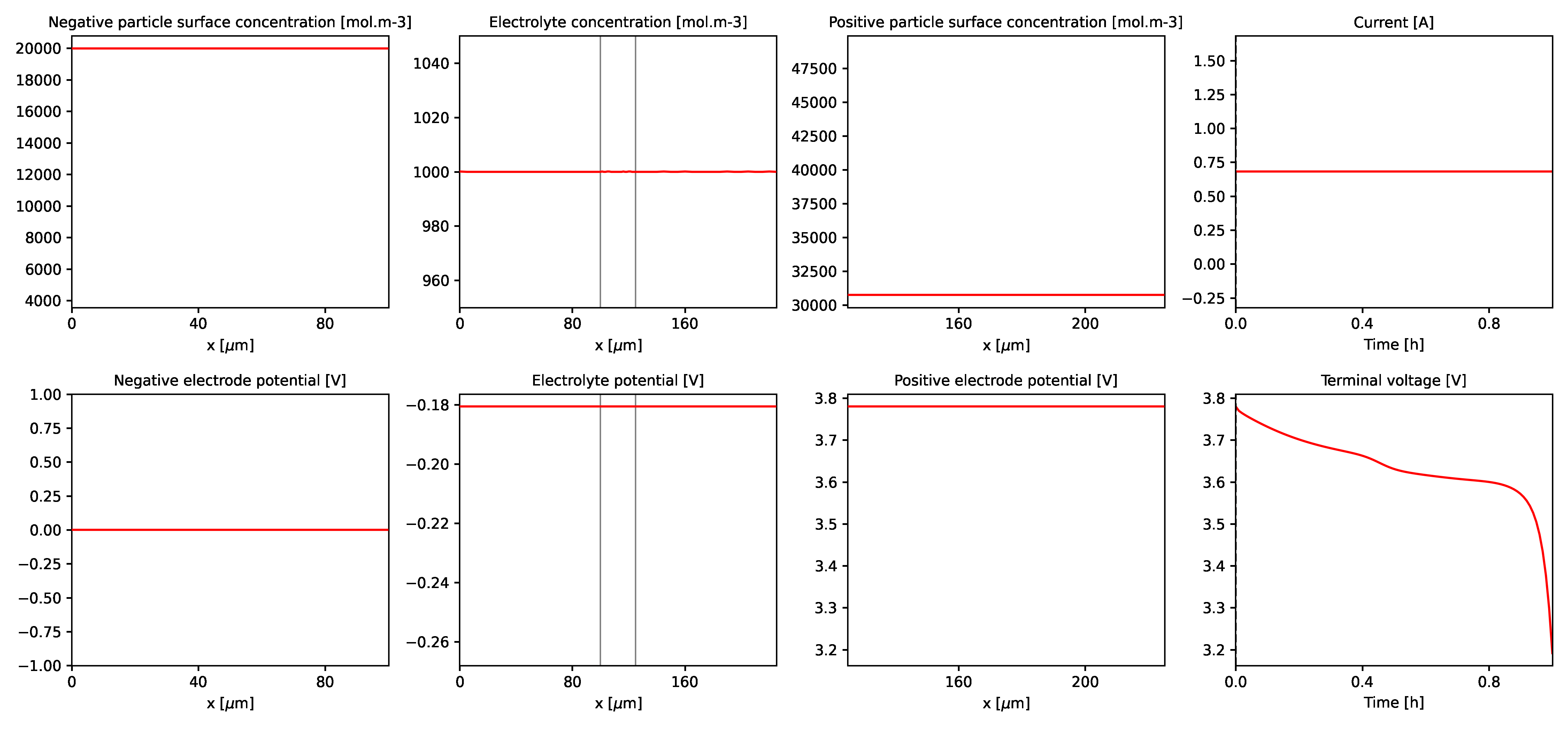Tip

An interactive online version of this notebook is available, which can be accessed via# A step-by-step look at the Simulation class#

The simplest way to solve a model is to use the Simulation class. This automatically processes the model (setting of parameters, setting up the mesh and discretisation, etc.) for you, and provides built-in functionality for solving and plotting. Changing things such as parameters in handled by passing options to the Simulation, as shown in the Getting Started guides, example notebooks and documentation.

In this notebook we show how to solve a model using a Simulation and compare this to manually handling the different stages of the process, such as setting parameters, ourselves step-by-step.

:

%pip install "pybamm[plot,cite]" -q    # install PyBaMM if it is not installed
import pybamm


## Simulation#

The easiest way to get started is to pick a model and create a simulation using that model. For simplicity, we’ll use the SPM with all the default options here.

:

model = pybamm.lithium_ion.SPM()
simulation = pybamm.Simulation(model)


The simulation can then be solved, passing a time interval (in seconds) to integrate over

:

simulation.solve([0, 3600])

:

<pybamm.solvers.solution.Solution at 0x2698c4bb3d0>


and the results plotted

:

simulation.plot()

:

<pybamm.plotting.quick_plot.QuickPlot at 0x2698c4da370>


Simple!

A GIF of the simulation can also be obtained

:

# using less number of images in the example
# for a smoother GIF use more images
simulation.create_gif(number_of_images=5, duration=0.2, output_filename="simulation.gif")


Displaying the GIF using markdown -## Processing the model step-by-step#

One way of gaining more control over the simulation processing is by passing options, as outlined in the documentation. However, you can also process the model step-by-step yourself. A detailed example of this can be found in the SPM notebook, but here we outline the basic steps.

First we pick a model

:

model = pybamm.lithium_ion.SPM()


Next we must set up the geometry. We’ll use the default geometry for the SPM. In all of the following steps we will also use the default settings provided by the model. For a look at changing these options, see the change settings notebook.

:

geometry = model.default_geometry


Both the model and geometry depend on parameters (such as the electrode thickness, or diffusivity). We’ll use the default model parameters

:

param = model.default_parameter_values


Now that we have picked our parameters we can “process” the model and geometry. This just means we look through the model and geometry for any parameter symbols and replace them with the numeric values (or functions, in the case of parameters that have functional dependence) defined by our parameter values.

:

param.process_model(model)
param.process_geometry(geometry)


Next we must create a mesh on which to solve the discretised equations. This not only depends on the geometry, but also on the type of submesh (e.g. uniformly space) and number of mesh points to use.

:

mesh = pybamm.Mesh(geometry, model.default_submesh_types, model.default_var_pts)


Now that we have defined a mesh we can discretise our model. In order to do so we must choose a spatial method. The default for the SPM is the Finite Volume Method. We first define a discretisation, which depends on the mesh and spatial method, and then use this to process our model. This turns the variables in the models into a StateVector, and replaces spatial operators with matrix-vector multiplications, ready to be passed to a time stepping algorithm.

:

disc = pybamm.Discretisation(mesh, model.default_spatial_methods)
disc.process_model(model)

:

<pybamm.models.full_battery_models.lithium_ion.spm.SPM at 0x2699d43f100>


Finally, we pick a solver to step the problem forward in time. We’ll use the default ODE solver for the SPM

:

solver = model.default_solver


We then integrate in time using the solve command, as with the simulation. Note that we now have to pass the model object to the solve command, and that we return the solution object so that we can interact with it later.

:

solution = solver.solve(model, [0, 3600])


We can create the default slider plot by passing the solution object to the dynamic_plot method

:

pybamm.dynamic_plot(solution)

:

<pybamm.plotting.quick_plot.QuickPlot at 0x2699064c760>